New update is available. Click here to update.
Last Updated: Mar 16, 2023

# Convolution layer, Padding, Stride, and Pooling in CNNAuthor Tashmit0 upvotes

## Introduction

In deep learning, a convolutional neural network is the artificial neural network, most commonly applied to analyze visual imagery. It is a primary category for image classification and recognition in neural networks. Some areas where convolutional neural networks are widely used are scene labeling, object detections, face recognition, etc.

## How CNN works

This neural network takes an image as input, classified, and processed under a specific category: dog, cat, lion, tiger, etc. The computer looks at the image as an array of pixels and depends on the resolution of the picture. Based on the image resolution, it will look at the image as height * width * dimension.

For example, consider an RGB image as a 5 * 5 * 2 array and the grayscale image as a 4 * 4 * 1 array. Each input image will go through a series of layers: convolution, padding, strides, and pooling. Post that, we will apply the Soft-max function to classify an object with probabilistic values 0 and 1.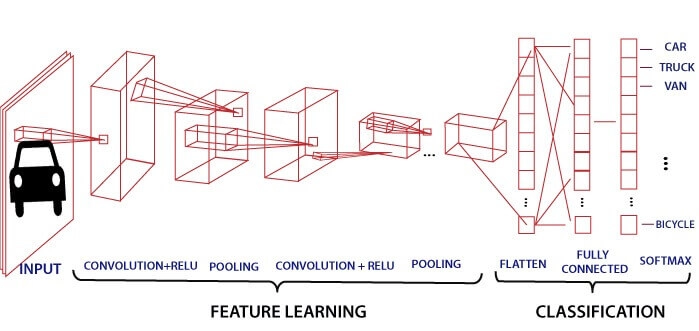## Convolution Layers

The Convolution Layers are the initial layers to pull out features from the image. It maintains the relationship between pixels by learning features using a small input data sequence. It is a mathematical term that takes two inputs, an image matrix and a kernel or filter. The result is calculated by: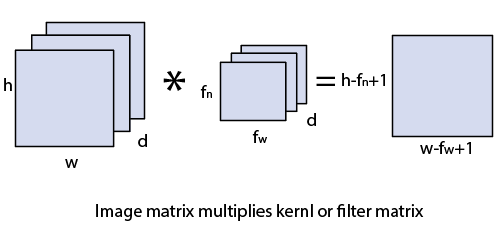In the above image,

The image matrix is h x w x d

The dimensions of the filter are fh x fw x d

The output is calculated as (h- fh +1)(w- fw+1) x 1

Now, let us take an example and solve a 5x5 image matrix whose pixel values are 0, 1 and the filter matrix as 3x3: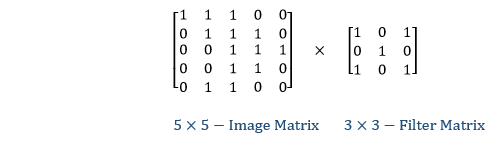The matrix multiplication will work as follows: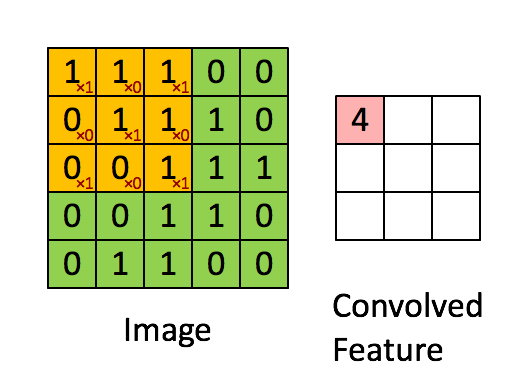The final convolution layers output matrix of a 5x5 image multiplied with a 3x3 filter will be: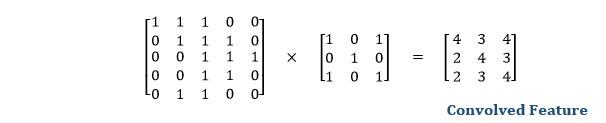The convolution of the image with different filter values can produce a blur or sharpened image. The size of the output image is calculated by:

(m-n+1)(m-n+1)

## Strides

When the array is created, the pixels are shifted over to the input matrix. The number of pixels turning to the input matrix is known as the strides. When the number of strides is 1, we move the filters to 1 pixel at a time. Similarly, when the number of strides is 2, we carry the filters to 2 pixels, and so on. They are essential because they control the convolution of the filter against the input, i.e., Strides are responsible for regulating the features that could be missed while flattening the image. They denote the number of steps we are moving in each convolution. The following figure shows how the convolution would work.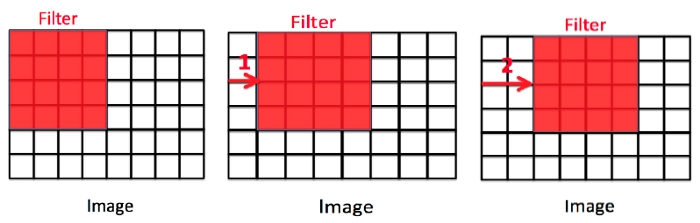In the first matrix, the stride = 0, second image: stride=2, and the third image: stride=2. The size of the output image is calculated by:

[{(n+2p-f+1)/s}+1][{(n+2p-f+1)/s}]

The padding plays a vital role in creating CNN. After the convolution operation, the original size of the image is shrunk. Also, in the image classification task, there are multiple convolution layers after which our original image is shrunk after every step, which we don’t want.

Secondly, when the kernel moves over the original image, it passes through the middle layer more times than the edge layers, due to which there occurs an overlap.

To overcome this problem, a new concept was introduced named padding. It is an additional layer that can add to the borders of an image while preserving the size of the original picture. For example: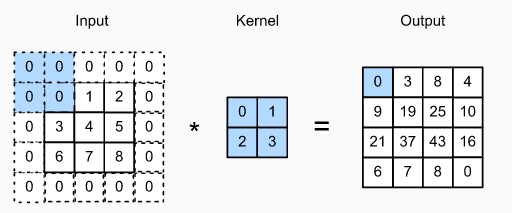So, if an n x n matrix is convolved with an ff matrix with a padding p, then the size of the output image will be:

(n+2p-f+1) x (n+2p-f+1)

## Pooling

The pooling layer is another building block of a CNN and plays a vital role in pre-processing an image. In the pre-process, the image size shrinks by reducing the number of parameters if the image is too large. When the picture is shrunk, the pixel density is also reduced, the downscaled image is obtained from the previous layers. Basically, its function is to progressively reduce the spatial size of the image to reduce the network complexity and computational cost. Spatial pooling is also known as downsampling or subsampling that reduces the dimensionality of each map but retains the essential features. A rectified linear activation function, or ReLU, is applied to each value in the feature map. Relu is a simple and effective nonlinearity that does not change the values in the feature map but is present because later subsequent pooling layers are added. Pooling is added after the nonlinearity is applied to the feature maps. There are three types of spatial pooling:

### 1. Max Pooling

Max pooling is a rule to take the maximum of a region and help to proceed with the most crucial features from the image. It is a sample-based process that transfers continuous functions into discrete counterparts. Its primary objective is to downscale an input by reducing its dimensionality and making assumptions about features contained in the sub-region that were rejected.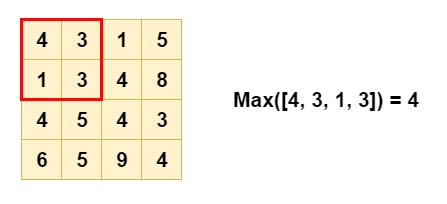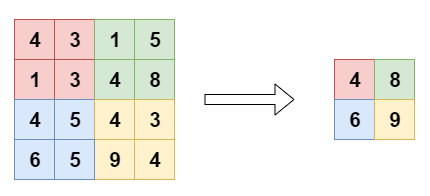### 2. Average Pooling

It is different from Max Pooling; it retains information about the lesser essential features. It simply downscales by dividing the input matrix into rectangular regions and calculating the average values of each area.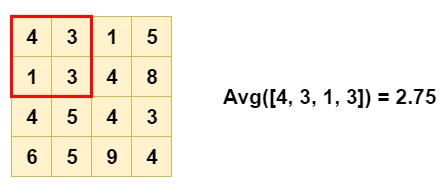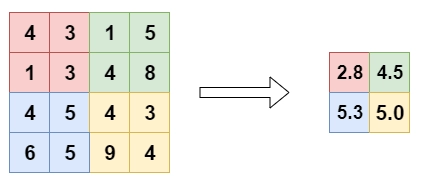### 3. Sum Pooling

It is similar to Max pooling, but instead of calculating the maximum value, we calculate the mean of each sub-region.

1. What are dropouts?
A dropout is an approach where randomly selected neurons are ignored during training; they are dropped out randomly. In other words, their contribution to the activation of downstream neurons is temporarily removed on the forward pass, and any weight updates are not applied to the neuron on the backward pass

2. How is convolution different from pooling?
The significant difference is that a convolution layer extracts features from the data matrix, whereas the pooling layer only downsamples the data matrix.

3. How many filters must a CNN have?
CNN does not learn with a single filter; they know through multiple features in parallel for a given input. For example, it is usual for a convolution layer to learn from 32 to 512 filters in parallel for a piece of shared information.

## Key Takeaways

CNN is the most commonly used algorithm for image classification. It detects the essential features in an image without any human intervention. In this article, we discussed how a convolution neural network works, the various layers in CNN, such as convolution layer, stride layer, Padding layer, and Pooling layer. If you’re interested in going deeper, Check out our industry-oriented machine learning course curated by our faculty from Stanford University and Industry experts.

Codekaze-June23 India's Biggest Tech Hiring Challenge is LIVE!Go on top